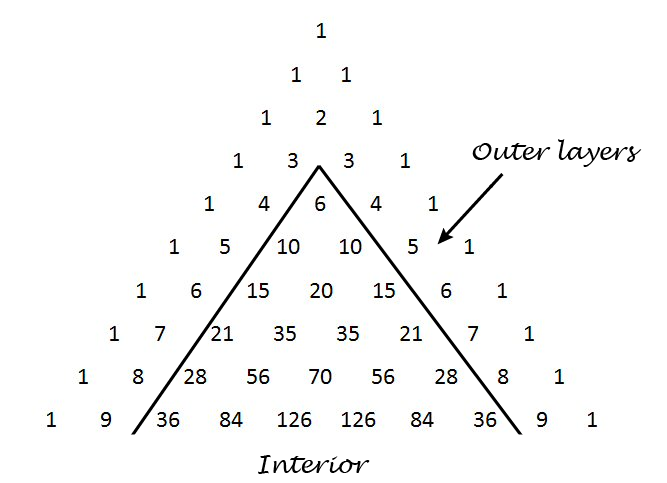# Distribution of numbers in Pascal’s triangle

This post explores a sentence from the book Single Digits:

Any number in Pascal’s triangle that is not in the outer two layers will appear at least three times, usually four.

Pascal’s triangle contains the binomial coefficients C(nr) where n ranges over non-negative numbers and r ranges from 0 to n. The outer layers are the elements with r equal to 0, 1, n-1, and n.We’ll write some Python code to explore how often the numbers up to 1,000,000 appear. How many rows of Pascal’s triangle should we compute? The smallest number on row n is C(n, 2). Now 1,000,000 is between C(1414, 2) and C(1415, 2) so we need row 1414. This means we need N = 1415 below because the row numbers start with 0.

I’d like to use a NumPy array for storing Pascal’s triangle. In the process of writing this code I realized that a NumPy array with `dtype int` doesn’t contain Python’s arbitrary-sized integers. This makes sense because NumPy is designed for efficient computation, and using a NumPy array to contain huge integers is unnatural. But I’d like to do it anyway, and the way to make it happen is to set `dtype` to `object`.

```    import numpy as np
from collections import Counter

N = 1415 # Number of rows of Pascal's triangle to compute

Pascal = np.zeros((N, N), dtype=object)
Pascal[0, 0] = 1
Pascal[1,0] = Pascal[1,1] = 1

for n in range(2, N):
for r in range(0, n+1):
Pascal[n, r] = Pascal[n-1, r-1] + Pascal[n-1, r]

c = Counter()
for n in range(4, N):
for r in range(2, n-1):
p = Pascal[n, r]
if p <= 1000000:
c[p] += 1
```

When we run this code, we find that our counter contains 1732 elements. That is, of the numbers up to one million, only 1732 of them appear inside Pascal’s triangle when we disallow the outer two layers. (The second layer contains consecutive integers, so every positive integer appears in Pascal’s triangle. But most integers only appear in the second layer.)

When Single Digits speaks of “Any number in Pascal’s triangle that is not in the outer two layers” this cannot refer to numbers that are not in the outer two layers because every natural number appears in the outer two layers. Also, when it says the number “will appear at least three times, usually four” it is referring to the entire triangle, i.e. including the outer two layers. So another way to state the sentence quoted above is as follows.

Define the interior of Pascal’s triangle to be the triangle excluding the outer two layers. Every number n in the interior of Pascal’s triangle appears twice more outside of the interior, namely as C(n, 1) and C(nn-1). Most often n appears at least twice in the interior as well.

This means that any number you find in the interior of Pascal’s triangle, interior meaning not in the two outer layers, will appear at least three times in the full triangle, usually more.

Here are the statistics from our code above regarding just the interior of Pascal’s triangle.

• One number, 3003, appears six times.
• Six numbers appear four times: 120, 210, 1540, 7140, 11628, and 24310.
• Ten numbers appear only once: 6, 20, 70, 252, 924, 3432, 12870,  48620, 184756, and 705432.
• The large majority of numbers, 1715 out of 1732, appear twice.

## 4 thoughts on “Distribution of numbers in Pascal’s triangle”

1. 3003 appears 8 times in total but the extra two times you missed are farther down from row 1415. And this is the only number known so far appearing 8 times. There’s no evidence that numbers appearing more than 8 times exist (but no proof has yet been found that they do not exist).

The smallest number that appears exactly 6 times in the whole triangle is 61,218,182,743,304,701,891,431,482,520. There are infinitely many such numbers but they are extremely sparse.

2. A small erratum to the above: 61,218,182,743,304,701,891,431,482,520 is the smallest number greater than 3003 that appears 6 or more times. Also, existence of an upper limit of multiplicity has been proved although the exact value is not known as of yet. It could be 8. It is most likely not greater than 12.

3. Owen Camp

yes 3003 appears eight times. twice in the integer rows, twice in the triangular # rows (row 78), and four times in the body of the triangle (rows 14 & 15)
120, 210, & 1540 (& others) appear six times in the Triangle twice in the integer rows, twice in the triangular # rows (rows 16, 21, & 56 ), and twice in the body of the triangle (rows 10 & 56)
Camp Conjecture #1: only 3003 appears eight times in the triangle. (If false a super computer can disprove it in minutes.)

4. Lee Mosher

xpil wrote that “existence of an upper limit on multiplicity has been proved”.

The existence of that upper limit was conjectured by Singmaster in a 1971 paper in the journal “Americal Mathematical Monthly”. As far as I can tell from a literature search, the conjecture has not yet been proved. The latest result I can find in the literature is a 2016 paper by Hugo Jenkins in the journal “Integers”, which contains a nice survey of the history of the problem.